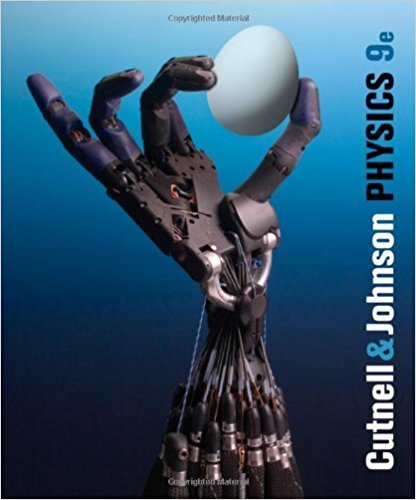×
×

# Solutions for Chapter 30: The Nature of the Atom## Full solutions for Physics, | 9th Edition

ISBN: 9780470879528Solutions for Chapter 30: The Nature of the Atom

Solutions for Chapter 30
4 5 0 411 Reviews
15
3
##### ISBN: 9780470879528

Physics, was written by and is associated to the ISBN: 9780470879528. This expansive textbook survival guide covers the following chapters and their solutions. Chapter 30: The Nature of the Atom includes 105 full step-by-step solutions. This textbook survival guide was created for the textbook: Physics,, edition: 9. Since 105 problems in chapter 30: The Nature of the Atom have been answered, more than 77553 students have viewed full step-by-step solutions from this chapter.

Key Physics Terms and definitions covered in this textbook
• //

parallel

• any symbol

average (indicated by a bar over a symbol—e.g., v¯ is average velocity)

• °C

Celsius degree

• °F

Fahrenheit degree

×